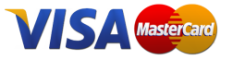﻿ Equivalency Cubes 51pc - Singapore MathsView larger

# Equivalency Cubes 51pc

R155.00 VAT incl.

Illustrating the relationship between fractions, decimals and percentage is a snap with this set of 51 colour-coded cubes.

### QuantityDurable, interlocking cubes help students visualise and understand fractions concepts in by building simple, visual mathematical models.  Cubes are marked with respective fractions, percentages and decimals.

CONTAINS
51 Pieces

 ISBN RGS6150

### 30 other products in the same category: